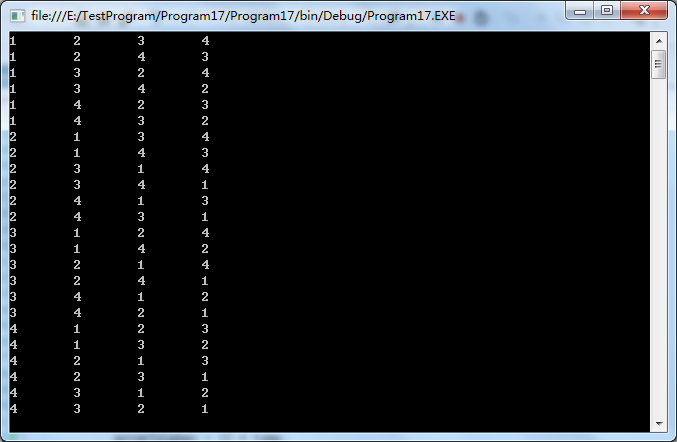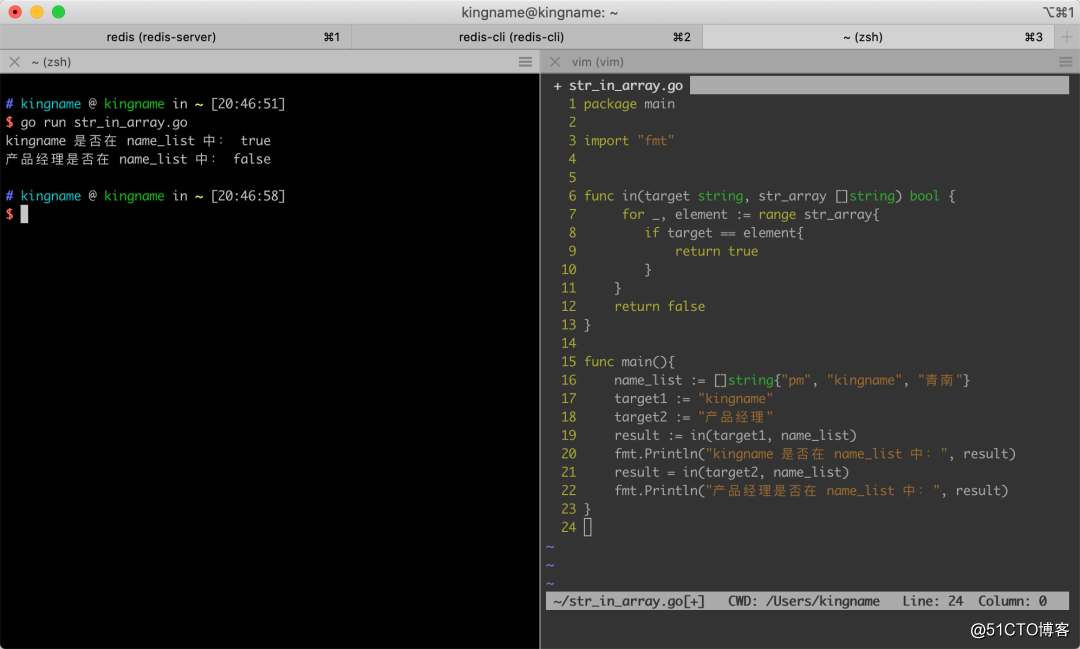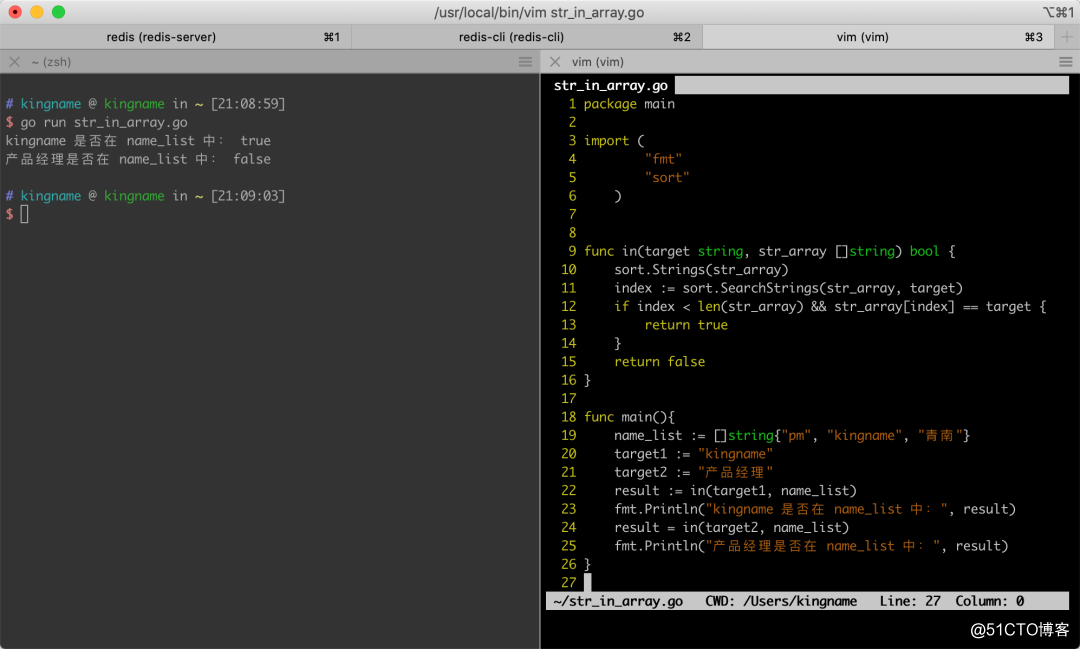• 判断n不在数组内
千次阅读
2017-12-27 09:09:00

判断某个值是否在某数组内的方法如下：

1.jQuery.inArray( value, array [, fromIndex ] )  ------  fromIndex( 数组索引值，表示从哪里在开始查找。默认值是0 )

描述：在数组中查找指定值并返回它的索引（如果没有找到，则返回-1）

inArray: function( elem, arr, i ) {
var len;
if ( arr ) {
if ( indexOf ) {
return indexOf.call( arr, elem, i );
}
len = arr.length;
i = i ? i < 0 ? Math.max( 0, len + i ) : i : 0;
for ( ; i < len; i++ ) {
// Skip accessing in sparse arrays
if ( i in arr && arr[ i ] === elem ) {
return i;
}
}
}
return -1;
},

$.inArray("b",["a","b","abc"]) ------ 1$.inArray("c",["a","b","abc"])  ------   -1

2.循环操作

function in_array(elem, arr) {
var i = 0, n = arr.length;
for (;i < n;++i)
if (arr[i] === elem)
return true;
return false;
}

in_array("b",["a","b","abc"])  ------   true
in_array("c",["a","b","abc"])  ------   false

3.正则操作

Array.prototype.in_array=function(elem){
var r=new RegExp(','+elem+',');
return r.test(','+this.join()+',');
};

[1,"d","abd","c"].in_array("1")  ------   true
[1,"d","abd","c"].in_array("ddd")  ------   false

4.indexOf()  ------  存在兼容性，IE中Array并没有这个方法

function in_array(elem,arr){
var str=','+arr.join(",")+",";
return str.indexOf(","+elem+",") != -1;
}

in_array("b",["a","b","abc"])  ------   true
in_array("c",["a","b","abc"])  ------   false

关于function in_array()和Array.prototype.in_array=function(){}这俩种形式都可以，一个是函数，另一个是类方法的扩展。

如果大家有更好的方法或者其他方法，可以留言发表自己的看法。

转载于:https://www.cnblogs.com/wjmm/p/8119285.html

更多相关内容
• python实现判断数组是否包含指定元素的方法本文实例讲述了python实现判断数组是否包含指定元素的方法。分享给大家供大家参考。具体如下：python判断数组是否包含指定的元素的方法，直接使用in即可，python真是简单...

python实现判断数组是否包含指定元素的方法

本文实例讲述了python实现判断数组是否包含指定元素的方法。分享给大家供大家参考。具体如下：

python判断数组是否包含指定的元素的方法，直接使用in即可，python真是简单易懂

print 3 in [1, 2, 3] # membership (1 means true

inventory = ["sword", "armor", "shield", "healing potion"]

if "healing potion" in inventory:

print "You will live to fight another day."

运行结果如下：

True

You will live to fight another day.

希望本文所述对大家的Python程序设计有所帮助。

时间： 2015-07-13

本文实例讲述了python获取元素在数组中索引号的方法.分享给大家供大家参考.具体如下: 这里python是通过index方法获取索引号的 li = ['a', 'b', 'new', 'D', 'z', 'example', 'new', 'two', 'elements'] print li.index("example") print li.index("new") print li.index("z") print "c&quo

一.isinstance() 在Python中可以使用type()与isinstance()这两个函数判断对象类型,而isinstance()函数的使用上比type更加方便. 复制代码 代码如下: # coding=utf-8   a = 10   def b():     pass   print isinstance(a,(int,str)) print isinstance(a,(float,str)) print isinstance(b,(str,int))   class c:

如: 复制代码 代码如下: a, b, c = b, c, a 来个复杂一点的例子,再来一顿家喻户晓的"冒泡排序"吧: 复制代码 代码如下: array = [1, 2, 5, 3, 6, 8, 4] for i in range(len(array) - 1, 1, -1): for j in range(0, i): if array[j] > array[j + 1]: array[j], array[j + 1] = array[j + 1], array[j] prin本文实例讲述了Python实现变量数值交换及判断数组是否含有某个元素的方法.分享给大家供大家参考,具体如下: 本来,这两个问题都属于的编程入门简单得不能再简单的问题,根本就不值得写篇记录来记录的. 一.变量数值交换 先说变量数值交换,从C语言开始,我们就知道要先设置一个临时变量,再把某元素的值覆盖此临时变量,避免临时覆盖等,如果不设置临时变量,还有位运算的交换形式 然而Python中根本就不用这么复杂,如果要交换变量e1,e2彼此的值,就下面一行代码就足矣: e1,e2=e2,e1; 比如,如下

Python追求简洁,诞生不少运算赋值规则,力求从简,其中就包括两个或者多个变量交换值. 普通语言中 复制代码 代码如下: # 声明变量 a=50 b=10 # 开始交换,先把其中一个值赋给临时变量,然后才能实现交换变量. tmp = a a = b b = tmp 在Python中,实现两个变量值交换非常方便 复制代码 代码如下: # 声明变量 a=50 b=10 # 开始交换变量 a,b = b,a 甚至可以多个变量同时交换 复制代码 代码如下: a=50 b=10 c=20 c,b,a =

解决的问题 需要将数组(list)或元组(tuple)中的元素导出到N个变量中. 解决的方案 任何序列都可以通过简单的变量赋值方式将其元素分配到对应的变量中,唯一的要求就是变量的数量和结构需要和序列中的结构完全一致. p = (1, 2) x, y = p # x = 1 # y = 2 data = ['google', 100.1, (2016, 5, 31)] name, price, date = data # name = 'google' # price = 100.1 # date

本文实例讲述了python实现数组插入新元素的方法.分享给大家供大家参考.具体如下: li=['a', 'b'] li.insert(0,"c") 输出为:['c', 'a', 'b'] li=['a', 'b'] li.insert(-1,"c") 输出为:[ 'a','c', 'b'] 希望本文所述对大家的Python程序设计有所帮助.

Json介绍 全名JavaScript Object Notation,是一种轻量级的数据交换格式.Json最广泛的应用是作为AJAX中web服务器和客户端的通讯的数据格式.现在也常用于http请求中,所以对json的各种学习,是自然而然的事情. 本文主要介绍的是利用Python判断变量是否为Json格式的字符串,对大家日常学习工作具有一定的参考价值,下面话不多说,直接来看代码吧. 示例代码如下 # -*- coding=utf-8 -*- import json def check_json_

PHP中有isset方法来检查数组元素是否存在,在Python中无对应函数. Python的编程理念是"包容错误"而不是"严格检查".举例如下: 复制代码 代码如下: Look before you leap (LBYL): if idx < len(array): array[idx] else: #handle this Easier to ask forgiveness than permission (EAFP): try: array[idx] ex

本文实例讲述了python简单获取数组元素个数的方法.分享给大家供大家参考.具体如下: 复制代码 代码如下: mySeq = [1,2,3,4,5]  print len(mySeq) 运行结果如下: 5 希望本文所述对大家的Python程序设计有所帮助.

Python判断变量是否已经定义是一个非常重要的功能,本文就来简述这一功能的实现方法. 其实Python中有很多方法可以实现判断一个变量是否已经定义了.这里就举出最常用的两种作为示例,如下所示: 方法一:try except方法: def isset(v): try : type (eval(v)) except : return 0 else : return 1 用法: if isset('user_name'): print 'user_name is defined' else prin

通过set()获取两个数组的交/并/差集: print set(a).intersection(set(b)) # 交集 print set(a).union(set(b)) # 并集 print set(a).difference(set(b)) # 差集,在a中但不在b中的元素 print set(b).difference(set(a)) # 差集,在b中但不在a中的元素 以上这篇Python 比较两个数组的元素的异同方法就是小编分享给大家的全部内容了,希望能给大家一个参考,也希望大家多多

方法一: 结合zip函数,使用map函数: List1 = [1,2,3,4] List2 = [5,6,7,8] List3 = map(lambda (a,b):a*b,zip(List1,List2)) print List3 方法二: 把列表转化为数组,使用np.multiply函数 List = [1,2,3] List2 = [5,6,7] List3 = np.multiply(np.array(List1),np.array(List2)) print List3.tolist(

本文实例讲述了python获得两个数组交集.并集.差集的房部分.分享给大家供大家参考.具体如下: 1. 获取两个list 的交集 #方法一: a=[2,3,4,5] b=[2,5,8] tmp = [val for val in a if val in b] print tmp #[2, 5] #方法二 print list(set(a).intersection(set(b))) 2. 获取两个list 的并集 print list(set(a).union(set(b))) 3. 获取两个

本文实例讲述了Python实现两个list对应元素相减操作.分享给大家供大家参考,具体如下: 两个list的对应元素操作,这里以相减为例: # coding=gbk v1 = [21, 34, 45] v2 = [55, 25, 77] #v = v2 - v1 # Error: TypeError: unsupported operand type(s) for -: 'list' and 'list' v = list(map(lambda x: x-x, zip(v2, v1)

Python DataFrame 如何设置列表字段/元素类型? 比如笔者想将列表的两个字段由float64设置为int64,那么就要用到DataFrame的astype属性,举例如图: 该例列表为"m_pred_survived"字段为"PassengerId"及"Survived",设置为int64类型,最后可以输出检验下是否正确. m_pred_survived = pd.DataFrame(columns=['PassengerId', '

本文介绍了java 两个数组合并的几种方法,分享给大家,也给自己留个笔记 需求:两个字符串合并(如果想去重复,参考下一篇--数组去重复及记录重复个数) //方法一 Arrays类 String[] a = {"A","B","C"}; String[] b = {"D","E"}; // List list = Arrays.asList(a); --OK // List<

javascript过滤数组重复元素的实现方法 以下是在网上找的资料,直接在项目中可以使用,大家可以参考下: 实现代码: function filterArray(receiveArray){ var arrResult = new Array(); //定义一个返回结果数组. for (var i=0; i本文实例讲述了C#求数组中元素全排列的方法.分享给大家供大家参考.具体如下: 1.算法描述 全排列的第一项是该数组的升序排列,最后一项是该数组的降序排列.本文中用到的了一个函数FindNextArray:从升序排列开始,不断使用函数FindNextArray,可以遍历全部排列,最终到达数组中元素的降序排列. FindNextArray函数的实现思路: 设有数组array为原数组的一个排列 1)找出数组的最大值 2)从后向前找:找到第一组array[i]>array[i-1]的数,以i位置为sig

jQuery 选择同时包含两个class的元素的实现方法 1. 交集选择: $(".a.b")--选择同时包含a和b的元素. 2. 并集选择:$(".a, .b")--选择包含a或者包含b的元素. 以上这篇jQuery 选择同时包含两个class的元素的实现方法就是小编分享给大家的全部内容了,希望能给大家一个参考,也希望大家多多支持我们.

在实际应用中,我们很多时候都可能需要去除数组中的重复元素,下面就是javascript数组去重的方法实现:

展开全文• 一日一技： Golang 中如何快速判断字符串是否一个数组中摄影：产品经理继续跟产品经理吃大餐使用 Python 的时候，如果要判断一个字符串是否另一个包含字符串的列表中，可以使用in 关键词，例如：name_list =...

一日一技：在 Golang 中如何快速判断字符串是否在一个数组中摄影：产品经理

继续跟产品经理吃大餐

在使用 Python 的时候，如果要判断一个字符串是否在另一个包含字符串的列表中，可以使用in 关键词，例如：

name_list = ['pm', 'kingname', '青南']

if 'kingname' in name_list:

print('kingname 在列表里面')

但是，Golang 是没有in这个关键词的，所以如果要判断一个字符串数组中是否包含一个特定的字符串，就需要一个一个对比：

package main

import "fmt"

func in(target string, str_array []string) bool {

for _, element := range str_array{

if target == element{

return true

}

}

return false

}

func main(){

name_list := []string{"pm", "kingname", "青南"}

target1 := "kingname"

target2 := "产品经理"

result := in(target1, name_list)

fmt.Println("kingname 是否在 name_list 中：", result)

result = in(target2, name_list)

fmt.Println("产品经理是否在 name_list 中：", result)

}

运行效果如下图所示：但这种方式有一个弊端，就是要遍历整个字符串数组。如果数组里面有100万条数据，那么平均要遍历50万次才能找到。这是一个非常费时间的操作。

有没有什么办法可以优化这个操作呢？

如果是有序的整型数组，那么我们可以使用二分查找，把时间复杂度O(n)降到对数时间复杂度。字符串能不能也这样操作呢？实际上是可以的。

在 Golang 中，有一个排序模块sort，它里面有一个sort.Strings()函数，可以对字符串数组进行排序。同时，还有一个sort.SearchStrings()函数，会用二分法在一个有序字符串数组中寻找特定字符串的索引。

结合两个函数，我们可以实现一个更高效的算法：

package main

import (

"fmt"

"sort"

)

func in(target string, str_array []string) bool {

sort.Strings(str_array)

index := sort.SearchStrings(str_array, target)

if index < len(str_array) && str_array[index] == target {

return true

}

return false

}

func main(){

name_list := []string{"pm", "kingname", "青南"}

target1 := "kingname"

target2 := "产品经理"

result := in(target1, name_list)

fmt.Println("kingname 是否在 name_list 中：", result)

result = in(target2, name_list)

fmt.Println("产品经理是否在 name_list 中：", result)

}

运行效果如下图所示：其中，sort.Strings是一个 in-place 的修改方式，是直接修改的 str_array。修改以后str_array变成有序的字符串数组。接下来通过二分查找快速定位。如果找到了，那么返回目标字符串在排序后的列表中第一次出现的索引。如果没有找到，那么返回数组中最后一个元素的索引。所以只要 index 小于最后一个元素的索引，那么目标字符串肯定存在；如果等于最后一个元素的索引，但是值不等于最后一个元素，那么目标字符串就不存在于字符串数组中。

通过先排序再查询的方式，对于100万个元素的字符串数组，只需要查询20次左右就能确认字符串是否存在。速度大大提升。

最后考大家一个思考题。name_list一开始是乱序的字符串数组，在上图第23行，如果打印一下 name_list，打印出来的是经过排序的，还是没有经过排序的字符串数字？

参考资料展开全文• 先上代码 public static void main (String []args){ int []c={1,5,6,7,9};... public static void panDuan(int n,int[]a){ boolean c=false; for (int b=0;b<a.length;b++){ ...

先上代码

public static void main (String []args){
int []c={1,5,6,7,9};
int d=5;
panDuan(d,c);
}
public static void panDuan(int n,int[]a){
boolean c=false;
for (int b=0;b<a.length;b++){

if(n==a[b]){
c=true;
System.out.println(b);
}

}
if(c==false){
System.out.println(n+"并没有在数组中出现过");
}

}
}


考虑数组中重复出现需要判断的整数情况,可能会多次打印下标,下标不会重复,所以可以写在循环内.
如果没有出现则只需输出一条语句,
加入判断条件来排除重复出现输出"并没有出现"语句.

public static void panDuan (int []arr,int n){
int b=0;
for(int a=0;a<arr.length;a++){
if(n==arr[a]){
b=1;
System.out.println(a);
}

}
if(b==0){
System.out.println("-1");
}
}
public static void main(String []args){
int []c={1,5,6,3,4};
int d=2;
panDuan(c,d);
}


同理加入数字定义判断 ,当数组中没有重复出现比较的数字时,运用这种判断方法隔离单一数字不同的输出情况,只保留数字n与数组arr全部不同的这种情况,再输出相关语句.

展开全文• 实际开发中，经常需要查询数组中的元素。例如，学校为每位同学分配了一个唯一的编号，现在有一个数组，保存了实验班所有同学的编号信息，如果有家长想知道他的孩子是否进入了实验班，只要提供孩子的编号就可以，...
• 使用快排每完成一轮排序，判断基准数的索引 和 数组长度-N 的关系 索引 = 数组长度-N ： 此基准数就是要找的第N大元素 索引 > 数组长度-N ： 要找的元素左边 索引 < 数组长度-N ： 要找的元素右边 import ...
• function contains() { local n=$# local value=${!n} for ((i=1;i < $#;i++)) { if [ "${!i}" == "${value}" ]; then echo "y" return 0 fi } ec... • 我基本上需要一个函数来检查字符串的字符(每个字符)是否在数组中.到目前为止,我的代码工作正常,但无论如何,这里是$allowedChars = array("a","b","c","d","e","f","g","h","i","j","k","l","m","n","o","p","q","r...
• 给定两个无序数组数组arr1[0…m-1] 和 数组arr2[0…n-1] ,判断数组arr2是否是arr1的子集。 比如 arr1[] = {11, 1, 13, 21, 3, 7} arr2[] = {11, 3, 7, 1} 输出true arr1[] = {1, 2, 3, 4, 5, 6}, arr2[] = {1, ...
• 写一个函数，判断某个数是否某个数组中 如果存在则返回其在数组中的位置，否则返回-1，主函数中调用 利用数组的下标法 给出已知的数组，读入一个数判断是否存在，若存在，找出在数组中的位置 /* Note:...
• ## Go语言数组详解

千次阅读 2021-01-17 17:02:04
因为数组的长度是固定的，所以Go语言中很少直接使用数组。和数组对应的类型是 Slice(切片)，Slice 是可以增长和收缩的动态序列，功能也更灵活，但是想要理解 slice 工作原理的话需要先理解数组，所以本节主要为...
• 题目：一个长度为n数组里的所有数字都0-n-1的 范围数组中某些数字是重复的，但是知道有几个数字重复了，也知道每个数字重复了几次。请找出数组中任意一个重复的数字。例如，如果输入长度为7的数组{2,3...
•  if [ "$y_n" == "$line" ]  then  return 1  break  else  continue  fi  ((i++)) done } test_array \$var array
• 1.isArray（）是Array类型的一个静态方法，可以用于判断一个值是否为数组 语法 Array.isArray(obj) 参数 obj :需要检测的值。 返回值 如果值是 Array，则为true; 否则为false。 举个例子 var a = [1,2,3]; ...
• 数组长度为n，数的范围{0，n-1}；数组元素随机排放，可能有重复值，怎样判断是否有重复元素？下面说明一下思路，这个问题各种办法都可以解决，但是算法的复杂度和性能上却各相同，最笨的办法就是逐个比较。 ...
• 判断一个数组是否为有序数组 #include<stdio.h> #include<stdlib.h> #include<string.h> #include<assert.h> bool Monoton(int* br, int n) { assert(br != nullptr && n > 1)...
• Matlab如何判断数组中是否为空数组、Matlab如何判断两个数组内容是否相等、Matlab如何检测数组中的元素是否为NaN（空值）、统计数组为NaN的元素的个数matlab
• 使用 Python 的时候，如果要判断一个字符串是否另一个包含字符串的列表中，可以使用in 关键词，例如：name_list=['pm','kingname','青南']if'kingname'inname_list:print('kingname列表里面')但是，Golang 是...
• 设二维数组a、b 含有m*n 个整数。写一个算法判断a,b两个数组中所有元素是否互不相同?输出相关信息 a={1,2,3,4,5,6,7,8,9,10,11,12}; b={1,2,3,3,5,6,7,8,9,10,11,12}; 运行结果截图： 代码： /*4.18 ...数据结构
• filter2、conv2报错：支持N数组 错误原因 对于彩色图像，imread函数返回一个3维数组，其中第三维度有3个元素分别为R、G和B。 即使你读取的是黑白图像，它其实还是RGB图像。怎么判断？ 读取一张图片： A=imread...matlab
• 直接用 if x in listA 看到一个更强悍的:y = [[1,2],3,4]x=1z=[i for i in y if ((type(i)==list) and (x in i)) or ((type(i) !=list) and (x==i)) ]...就是查找X哪个list的元素,看看那一句,简直就是自然语言...python
• 做第三个题目的时候，整个人的思路卡住了，因为if（）函数里面能嵌套for()循环，然后如果for()函数里面嵌套了if_else()的判断思路，则需要进行每一次的数组元素判断，这样打印输出的结果是每一次判断的结果，...数据结构
• 自定义一个数组a，读入一个整数n，如果n在数组中存在，则输出n的下标，存在输出-1 import java.util.Scanner; public class TestArray { public static void main(String[] args) { Scanner sc = new Scanner...java
• 总体思路：先对数组排序，然后去掉重复元素，对比两个去重以后的数组长度是否相同，若不同则两个数组不一致，若相同，则依次判断两个数组对应的元素是否相同，若出现一处不同，则不一致。 #include <stdio.h>...排序算法
• 匿名用户1级2018-11-18 回答PHP中求数组的交集，我们可以与PHP给我们提供的现成函数：array_intersect()，其用法格式为：array array_intersect(array array1,array array2[,arrayN…])根据上述的语法格式，我们来...mysql 数组判断
• 一个长度为N的整形数组数组中每个元素的取值范围是[0,N-1]，写一个算法判断数组中是否存在重复的数字数据结构 排序算法
• Golang 如何判断数组某个元素是否存在(isset),数值,方法,结构,是否存在,判断是否Golang 如何判断数组某个元素是否存在(isset)易采站长站，站长之家为您整理了Golang 如何判断数组某个元素是否存在(isset)的相关内容...
• 各种类型字符数组的大小 #include <stdio.h> #include <stdlib.h> int main() { //只要sizeof（数组名）... printf("%d\n", sizeof(arr));//sizeof计算数组的大小，char类型为一个字节，6*1=6 printf(指针 数据结构 sizeof
• sizeof计算数组大小 2、案例...